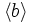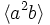# SmallGroup(16,3)

View a complete list of particular groups (this is a very huge list!)[SHOW MORE]

## Definition

This group can be defined using the following presentation:$G := \langle a,b,c \mid a^4 = b^2 = c^2 = e, ab = ba, bc = cb, cac^{-1} = ab \rangle$

Note that$G$ is generated by$a,c$ alone, because the final relation allows us to write$b$ in terms of$a$ and$c$.

The subgroup$\langle a,b \rangle$ is isomorphic to the direct product of Z4 and Z2, and the element$c$ is an element of order two that acts on the subgroup$\langle a,b \rangle$ by conjugation by fixing$b$ and sending$a$ to$ab$.

## Group properties

Want to compare with other groups of the same order? Check out groups of order 16#Group properties.
Property Satisfied Explanation
abelian group No$a,c$ don't commute
group of prime power order Yes
nilpotent group Yes prime power order implies nilpotent
group of nilpotency class two Yes
supersolvable group Yes
T-group No The subgroup$\langle a \rangle$ is 2-subnormal, not normal
monolithic group No
ambivalent group No
rational group No
rational-representation group No

## Subgroups

Further information: subgroup structure of SmallGroup(16,3)

1. The trivial group. (1)
2. The subgroup$\langle b \rangle$, which is the unique characteristic subgroup of order$2$. Isomorphic to cyclic group:Z2. It is the commutator subgroup, and can also be described as the unique group of order two containing an element that is not a square but is a product of squares. The quotient group is isomorphic to direct product of Z4 and Z2. (1)
3. The subgroups$\langle a^2 \rangle$ and$\langle a^2b \rangle$, which are both normal subgroups related by an outer automorphism. Isomorphic to cyclic group:Z2. The quotient group for each is isomorphic to dihedral group:D8. (2)
4. The subgroups$\langle c \rangle$,$\langle bc \rangle$,$\langle a^2c \rangle$ and$\langle a^2bc \rangle$. Neither is normal, and they come in two conjugacy classes of size two each. Isomorphic to cyclic group:Z2. (4)
5. The subgroup$\langle a^2,b \rangle$, which is the center, first agemo subgroup, and Frattini subgroup. Isomorphic to Klein four-group. The quotient group is isomorphic to Klein four-group. (1)
6. The subgroups$\langle b,c \rangle$ and$\langle b, a^2c\rangle$. Both are normal subgroups and are related by an outer automorphism. Isomorphic to Klein four-group. The quotient group is isomorphic to cyclic group:Z4. (2)
7. The subgroups$\langle a^2,c \rangle$,$\langle a^2,bc \rangle$,$\langle a^2b,c\rangle$, and$\langle a^2b, bc \rangle$. Two conjugacy classes of size two each. Isomorphic to Klein four-group. The quotient group is also isomorphic to a Klein four-group. (4)
8. The subgroups$\langle a \rangle$,$\langle ab \rangle$,$\langle ac \rangle$, and$\langle abc \rangle$. All of them are related by outer automorphisms, and they form two conjugacy classes of subgroups of size two each:$\langle a \rangle$ is conjugate to$\langle ab \rangle$, while$\langle ac \rangle$ is conjugate to$\langle abc \rangle$. Isomorphic to cyclic group:Z4. (4)
9. The subgroups$\langle a,b \rangle$ and$\langle ac, b \rangle$. These are both normal subgroups related by an outer automorphism. Isomorphic to direct product of Z4 and Z2. The quotient group is isomorphic to cyclic group:Z2. (2)
10. The subgroup$\langle a^2,b,c \rangle$. Isomorphic to elementary abelian group:E8. The quotient group is isomorphic to cyclic group:Z2. (1)
11. The whole group. (1)

## GAP implementation

### Group ID

This finite group has order 16 and has ID 3 among the groups of order 16 in GAP's SmallGroup library. For context, there are 14 groups of order 16. It can thus be defined using GAP's SmallGroup function as:

SmallGroup(16,3)

For instance, we can use the following assignment in GAP to create the group and name it$G$:

gap> G := SmallGroup(16,3);

Conversely, to check whether a given group$G$ is in fact the group we want, we can use GAP's IdGroup function:

IdGroup(G) = [16,3]

or just do:

IdGroup(G)

to have GAP output the group ID, that we can then compare to what we want.

### Other descriptions

The group can be constructed using the following GAP commands:

gap> F := FreeGroup(3);
<free group on the generators [ f1, f2, f3 ]>
gap> G := F/[F.1^4, F.2^2, F.1*F.2*F.1^(-1)*F.2^(-1),F.3^2,F.3*F.2*F.3^(-1)*F.2^(-1),F.3*F.1*F.3^(-1)*F.2^(-1)*F.1^(-1)];
<fp group on the generators [ f1, f2, f3 ]>